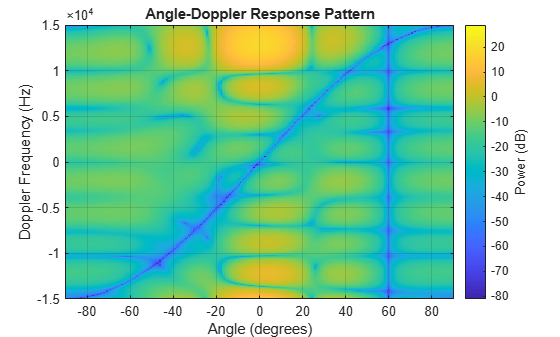# step

Package: phased

Perform ADPCA processing on input data

## Syntax

```Y = step(H,X,CUTIDX) Y = step(H,X,CUTIDX,ANG) Y = step(H,X,CUTIDX,DOP) Y = step(H,X,CUTIDX,PRF) [Y,W] = step(___) ```

## Description

Note

Starting in R2016b, instead of using the `step` method to perform the operation defined by the System object™, you can call the object with arguments, as if it were a function. For example, `y = step(obj,x)` and `y = obj(x)` perform equivalent operations.

`Y = step(H,X,CUTIDX)` applies the ADPCA pulse cancellation algorithm to the input data `X`. The algorithm calculates the processing weights according to the range cell specified by `CUTIDX`. This syntax is available when the `DirectionSource` property is `'Property'` and the `DopplerSource` property is `'Property'`. The receiving mainlobe direction is the `Direction` property value. The output `Y` contains the result of pulse cancellation either before or after Doppler filtering, depending on the `PreDopplerOutput` property value.

`Y = step(H,X,CUTIDX,ANG)` uses `ANG` as the receiving main lobe direction. This syntax is available when the `DirectionSource` property is `'Input port'` and the `DopplerSource` property is `'Property'`.

`Y = step(H,X,CUTIDX,DOP)` uses `DOP` as the targeting Doppler frequency. This syntax is available when the `DopplerSource` property is ```'Input port'```.

`Y = step(H,X,CUTIDX,PRF)` uses `PRF` as the pulse repetition frequency. This syntax is available when the `PRFSource` property is `'Input port'`.

```[Y,W] = step(___)``` also returns the processing weights, `W`. This syntax is available when the `WeightsOutputPort` property is `true`.

Note

The object performs an initialization the first time the object is executed. This initialization locks nontunable properties and input specifications, such as dimensions, complexity, and data type of the input data. If you change a nontunable property or an input specification, the System object issues an error. To change nontunable properties or inputs, you must first call the `release` method to unlock the object.

## Input Arguments

 `H` Pulse canceller object. `X` Input data. `X` must be a 3-dimensional M-by-N-by-P numeric array whose dimensions are (range, channels, pulses). You can specify this argument as single or double precision. The size of the first dimension of the input matrix can vary to simulate a changing signal length. A size change can occur, for example, in the case of a pulse waveform with variable pulse repetition frequency. `CUTIDX` Range cell. You can specify this argument as single or double precision. `PRF` Pulse repetition frequency specified as a positive scalar. To enable this argument, set the `PRFSource` property to ```'Input port'```. You can specify this argument as single or double precision. Units are in Hertz. `ANG` Receiving main lobe direction. `ANG` must be a 2-by-1 vector in the form `[AzimuthAngle; ElevationAngle]`, in degrees. The azimuth angle must be between –180 and 180. The elevation angle must be between –90 and 90. You can specify this argument as single or double precision. Default: `Direction` property of `H` `DOP` Targeting Doppler frequency in hertz. `DOP` must be a scalar. You can specify this argument as single or double precision. Default: `Doppler` property of `H`

## Output Arguments

 `Y` Result of applying pulse cancelling to the input data. The meaning and dimensions of `Y` depend on the `PreDopplerOutput` property of `H`: If `PreDopplerOutput` is `true`, `Y` contains the pre-Doppler data. `Y` is an M-by-(P–1) matrix. Each column in `Y` represents the result obtained by cancelling the two successive pulses.If `PreDopplerOutput` is `false`, `Y` contains the result of applying an FFT-based Doppler filter to the pre-Doppler data. The targeting Doppler is the `Doppler` property value. `Y` is a column vector of length M. `W` Processing weights the pulse canceller used to obtain the pre-Doppler data. The dimensions of `W` depend on the `PreDopplerOutput` property of `H`: If `PreDopplerOutput` is `true`, `W` is a 2N-by-(P-1) matrix. The columns in `W` correspond to successive pulses in `X`.If `PreDopplerOutput` is `false`, `W` is a column vector of length (N*P).

## Examples

expand all

Process a radar data cube using an ADPCA processor. Weights are calculated for the 71st cell of the data cube. Load the data cube from `STAPExampleData.mat`. Quantize the weights to 4 bits. Set the look direction to (0,0) degrees and the Doppler shift to 12.980 kHz.

```load STAPExampleData; sADPCA = phased.ADPCACanceller('SensorArray',STAPEx_HArray,... 'PRF',STAPEx_PRF,... 'PropagationSpeed',STAPEx_PropagationSpeed,... 'OperatingFrequency',STAPEx_OperatingFrequency,... 'NumTrainingCells',100,... 'WeightsOutputPort',true,... 'DirectionSource','Input port',... 'DopplerSource','Input port',... 'NumPhaseShifterBits',4); [y,w] = step(sADPCA,STAPEx_ReceivePulse,71,[0; 0],12.980e3); sAngDop = phased.AngleDopplerResponse(... 'SensorArray',sADPCA.SensorArray,... 'OperatingFrequency',sADPCA.OperatingFrequency,... 'PRF',sADPCA.PRF,... 'PropagationSpeed',sADPCA.PropagationSpeed); plotResponse(sAngDop,w);```